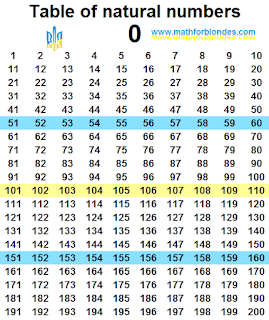## 7.16.2010

### Table of natural numbers

Table of natural numbers from 1 (one) to 200 (two hundred) is a sequence of positive integers which in mathematics it is accepted to name natural numbers. Here you can get the table of natural numbers free of charge. In a table the natural row of numbers is presented from 1 (units) to 200 (two hundred).

Attention! Zero is a natural number! In Russian mathematics a zero is not a natural number.Table of natural numbers

The sequence of natural numbers, formative the natural row of numbers is evidently presented in a table. Can this table bravely be named Periodic System of Natural Numbers from Nikolay khyzhnjak))) Why is my row of natural numbers closed on a number 120? Simply to me, as to any respecting itself cat, it was to write laziness farther. If you need the table of natural numbers of largenesses (well, there, to cover a settee) vitally, write in the comments ordering from sizes - I will execute certainly. To the first pussy-cat - free of charge)))

The table of natural numbers will help you masterly to determine the amount of different numbers in natural numbers and satisfy other mathematical needs. The table of natural numbers is worked out in the secret laboratories of extraterrestrial civilizations specially for blondes, in case of cruel interrogations inquisitors from mathematics))) are Presently examine the question of creation of table of natural numbers for a flush-off on mobile telephones (such to itself mathematical body armour).

To get the table of figure (numerals) from 1 to 20 - the table of natural numbers is here presented only, but not figure (numerals). Figure (numerals) exists only ten.

Natural figure (numerals) - numerals are Arabic, Roman et al, and natural are numbers.

Figure (numerals) 100 - one hundred is a number and this number 100 consists of three figure (numerals) is one unit 1 and two zeros 0 and 0. All together these three figure (numerals) form a number 100 - one hundred.

Draw a table of values for the first 6 natural numbers.